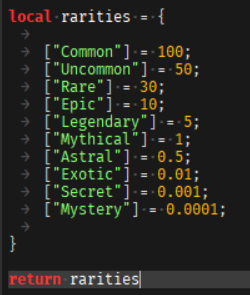# Problem with Pet System

Hello!

So i’m currently working on a Pet System for my fanbase on YouTube, but i’m having an issue.
When I buy a pet, it somehow gives me a random number of pets.
Sometimes it works perfectly, so it gives me 1 pet as intended, but sometimes it gives me 2 or 3.

Any idea?

``````local rep = game:GetService("ReplicatedStorage")

local remotes = rep:WaitForChild("Remotes")

local petsFolder = rep:WaitForChild("Pets")

local rarities = require(script.Parent:WaitForChild("Rarities"))

------------------------------------------------------

local character = player.Character
local humanoidRootPart = character:WaitForChild("HumanoidRootPart")

local random = math.random(0,100)
local pets = petsFolder:FindFirstChild(egg)

end

local function ChooseRandomPet(rarity)
local petNum = pets:FindFirstChild(rarity):GetChildren()
local pet = petNum[math.random(1, #petNum)]:Clone()
print(pet.Name)
pet.Parent = workspace
pet.CFrame = humanoidRootPart.CFrame
end

if random <= rarities.Common then
ChooseRandomPet("Common")
end

if random <= rarities.Uncommon then
ChooseRandomPet("Uncommon")
end

if random <= rarities.Rare then
ChooseRandomPet("Rare")
end

if random <= rarities.Epic then
ChooseRandomPet("Epic")
end

if random <= rarities.Legendary then
ChooseRandomPet("Legendary")
end

end

end

end)
``````
1 Like

im guessing that the rarities are number values?
It could be that it chose a random value between two rarities so it gave you two, im not sure though, could you give more info though?

1 Like

The rarities are values inside a module script
If you want the module script I can provide it2 Likes

on the first four rarities, put a print
something like this, for testing purposes.

``````if random <= rarities.Uncommon then
ChooseRandomPet("Uncommon")
print("!")
end
``````

i think it somehow chooses more than one rarity, but i could be wrong.

1 Like

I tried printing, and think you’re right. It’s printing ("!") two times when I hatch 1 egg.

1 Like

Try replacing all of the “if random <= rarity then” statements with this.

``````local RaritiesForThisEgg = {{"Common",rarities.Common},{"Uncommon",rarities.Uncommon},{"Rare",rarities.Rare},{"Epic",rarities.Epic},{"Legendary",rarities.Legendary}}
table.sort(RaritiesForThisEgg,function(i,i2)
return i2 > i
end)

local stop = false
for i = #RaritiesForThisEgg,1,-1 do
if stop == false then
local v = RaritiesForThisEgg[i]
if random <= v then
ChooseRandomPet(v)
print("horray!")
stop = true
end
end
end
``````

edit: Only have this one time, it should take care of every rarity.

1 Like

``````if random <= rarities.Uncommon then
ChooseRandomPet("Uncommon")
end
``````

try and do

``````if random <= rarities.Uncommon and random > rarities.Rare then
ChooseRandomPet("Uncommon")
end
``````

keep on doing this with every line, so if its epic, check if its higher than Legendary, and so on., i think that should work but i dont know.

1 Like

So now it gives me 1 pet, which is good. But it looks like it’s only giving me the Dog?

This comment is the solution. Thanks.

I tried @MasterStudioWorld 's comment but it was only giving me the Common pet.
Yours worked perfectly!

Thanks again @KoneGFX !

1 Like

No problem!thirty letters